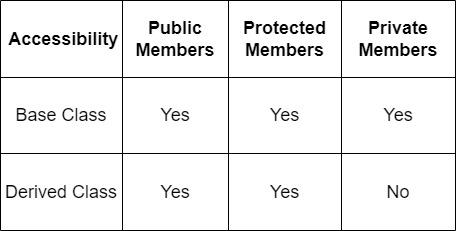Open in App
Not now

# C++ Inheritance Access

• Last Updated : 27 Nov, 2022

Prerequisites:

Before learning about Inheritance Access we need to know about access specifiers. There are three Access specifiers in C++. These are:

public – members are accessible from outside the class, and members can be accessed from anywhere.
private – members cannot be accessed (or viewed) from outside the class, i.e members are private to that class only.
protected – members cannot be accessed from outside the class, but, they can be accessed in inherited classes or derived classes.

### Public, Protected, and Private inheritance in C++

public, protected, and private inheritance have the following features:

• public inheritance makes public members of the base class public in the derived class, and the protected members of the base class remain protected in the derived class.
• protected inheritance makes the public and protected members of the base class protected in the derived class.
• private inheritance makes the public and protected members of the base class private in the derived class.

Accessibility Of Inheritance Access:Inheritance Access

## 1. C++ public Inheritance

In this example, public inheritance is demonstrated. Since private and protected members will not be directly accessed from main( ) so we have had to create functions name getPVT( ) to access the private variable and getProt( ) to access the protected variable from the inherited class.

Example:

## C++

 `// C++ program to demonstrate the working of public ` `// inheritance ` `#include ` `using` `namespace` `std; ` ` `  `class` `Base { ` `private``: ` `    ``int` `pvt = 1; ` ` `  `protected``: ` `    ``int` `prot = 2; ` ` `  `public``: ` `    ``int` `pub = 3; ` ` `  `    ``// function to access private member ` `    ``int` `getPVT() { ``return` `pvt; } ` `}; ` ` `  `class` `PublicDerived : ``public` `Base { ` `public``: ` `    ``// function to access protected member from Base ` `    ``int` `getProt() { ``return` `prot; } ` `}; ` ` `  `int` `main() ` `{ ` `    ``PublicDerived object1; ` `    ``cout << ``"Private = "` `<< object1.getPVT() << endl; ` `    ``cout << ``"Protected = "` `<< object1.getProt() << endl; ` `    ``cout << ``"Public = "` `<< object1.pub << endl; ` `    ``return` `0; ` `}`

Output

```Private = 1
Protected = 2
Public = 3```

## 2. C++ protected Inheritance

We know that protected members can only be accessed from the Derived class. These members cannot be directly accessed from outside the class. So we cannot use getPVT() from ProtectedDerived.This is also why we need to create getPub() function in the Derived class in order to access the pub variable.

Example:

## C++

 `// C++ program to demonstrate the working ` `// of protected inheritance ` `#include ` `using` `namespace` `std; ` ` `  `class` `Base { ` `private``: ` `    ``int` `pvt = 1; ` ` `  `protected``: ` `    ``int` `prot = 2; ` ` `  `public``: ` `    ``int` `pub = 3; ` ` `  `    ``// function to access private member ` `    ``int` `getPVT() { ``return` `pvt; } ` `}; ` ` `  `class` `ProtectedDerived : ``protected` `Base { ` `public``: ` `    ``// function to access protected member from Base ` `    ``int` `getProt() { ``return` `prot; } ` ` `  `    ``// function to access public member from Base ` `    ``int` `getPub() { ``return` `pub; } ` `}; ` ` `  `int` `main() ` `{ ` `    ``ProtectedDerived object1; ` `    ``cout << ``"Private cannot be accessed."` `<< endl; ` `    ``cout << ``"Protected = "` `<< object1.getProt() << endl; ` `    ``cout << ``"Public = "` `<< object1.getPub() << endl; ` `    ``return` `0; ` `}`

Output

```Private cannot be accessed.
Protected = 2
Public = 3```

## 3. C++ private Inheritance

We know that private members cannot be accessed from the Derived class. These members cannot be directly accessed from outside the class. So we cannot use getPVT() from PrivateDerived. So we need to create the getPub() function in PrivateDerived in order to access the pub variable.

Example:

## C++

 `// C++ program to demonstrate the working ` `// of private inheritance ` `#include ` `using` `namespace` `std; ` ` `  `class` `Base { ` `private``: ` `    ``int` `pvt = 1; ` ` `  `protected``: ` `    ``int` `prot = 2; ` ` `  `public``: ` `    ``int` `pub = 3; ` ` `  `    ``// function to access private member ` `    ``int` `getPVT() { ``return` `pvt; } ` `}; ` ` `  `class` `PrivateDerived : ``private` `Base { ` `public``: ` `    ``// function to access protected member from Base ` `    ``int` `getProt() { ``return` `prot; } ` ` `  `    ``// function to access private member ` `    ``int` `getPub() { ``return` `pub; } ` `}; ` ` `  `int` `main() ` `{ ` `    ``PrivateDerived object1; ` `    ``cout << ``"Private cannot be accessed."` `<< endl; ` `    ``cout << ``"Protected = "` `<< object1.getProt() << endl; ` `    ``cout << ``"Public = "` `<< object1.getPub() << endl; ` `    ``return` `0; ` `}`

Output

```Private cannot be accessed.
Protected = 2
Public = 3```

My Personal Notes arrow_drop_up
Related Articles